# Arrays in Excel VBA

Table of Contents

# VBA Arrays – basic informations

## What are arrays in VBA Excel – VBA Arrays

In this chapter of the VBA course you will learn what VBA arrays are and how to use them in data analysis. VBA arrays are blocks for storing data of the same type in an organized order. You can refer to each element of an array using its indexed address. Arrays can also be compared to variables that store other variables

Using arrays you have access to data in VBA in an easy and quick way without having to load them from the spreadsheet. Arrays in Excel VBA can be grouped into types depending on the number of array’s dimensions. Each element of the array can store data:

• One-dimensional array (list)
• Two-dimensional arrays (chessboard)
• Three-dimensional arrays (cube)
• Multidimensional arrays (for more than 3 dimensions, up to 60 dimensions)

You should declare array in Excel VBA when you want to use it in your code. After the declaration, you can assign elements to them. Below you can see types of VBA arrays. Multidimensional arrays are hard to visualize, so the way they are displayed is abstract.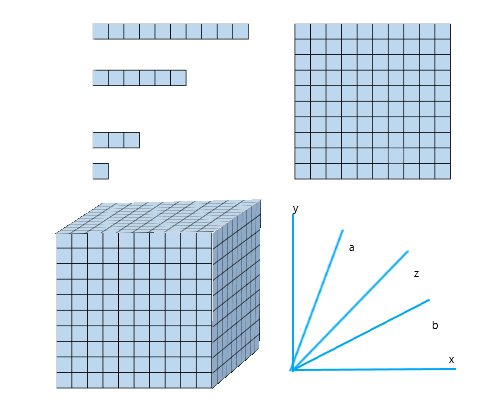Excel VBA Arrays – Dimensions

## Declaring and addressing elements of the array in VBA

Arrays in VBA should be declared. Arrays like VBA variables  have a data type. The data type for all elements can also be declared. If you don’t do this, the data will get the Variant type. It is important that all elements of the array have the same data type. You can make the declaration in several equivalent ways.

### Declaring an array with total number of elements:

By declaring an array in this way, you specify the maximum number of elements that array can accept. The most important thing with this declaration is that you specify the index of the last element in the array in brackets. VBA arrays are numbered by default from zero. So, if you want to declare an example of a one-dimensional array with a number of 10 elements, you should write it in this way Dim Name(9) . Then you refer to numbers from 0 to 9 when you want to use it.

```Sub arrayDeclarationSimpe()
'OfficeInside.Org

`'declaration of one-dimensional, 11 elements array without data-type declaration `
`Dim array1(10) `

`'declaration of three-dimensional, 11 elements array with data-type declaration `
`Dim array2(10, 10, 10) As String`

End Sub```

### Declaring an array with the specification indexes of elements:

Declaring an array with the index specification consists in determining the number of the first and last element of each dimension, ie. 1 to 12 or 2000 to 2005. Declaring with the index number determines the use of elements of the array significantly. You can give the index number on the example of the number of the year, month, day of the week, etc.

```Sub arrayDeclarationIndexes()
'OfficeInside.Org

'declaration of one-dimensional, 10 elements array without data-type declaration
Dim TabTablica1(1 To 10)

'declaration of three-dimensional, 11 elements array with data-type declaration
Dim TabTablica2(2017 To 2025, 1 To 12, 1 To 7) As String
End Sub```

### Mixed declaration of an array

You can also declare arrays in a mixed way. In the declaration, you use both previously learned ways.

```Sub arrayDeclarationMixed()
'OfficeInside.Org

'declaration of three-dimensional, with data-type declaration:
Dim TabTablica2(2017 To 2025, 10) As String
End Sub```

### Change in the number of elements in array

In VBA Excel, you can also declare an array without specifying the number of elements and dimensions that it should have. An attempt to enter data into it will return an error. To avoid the error, the number of elements in the array should be specified using the ReDim command, as in the example written below. You can also enlarge your array with new elements.

```Sub arrayDeclarationRedim()
'OfficeInside.Org

Dim TabTable()
ReDim TabTable(1 To 12, 6)
ReDim TabTable(1 To 50, 10, 2015 To 2030)
End Sub```

### Addressing array elements

Once an array is declared, you can enter data into it and read it . To perform these activities, you should address elements of the array. You need to use its indexes. To the elements of declared arrays, in order to write or read data, you can do it with following way:  ArrayName(index_number).

``````Sub arrayAddress()
'OfficeInside.Org
Dim arr(10) As String

arr(0) = "Excel"
arr(1) = "VBA"
arr(2) = "Tutorial"

Debug.Print arr(0)
Debug.Print arr(1)
Debug.Print arr(2)
End Sub``````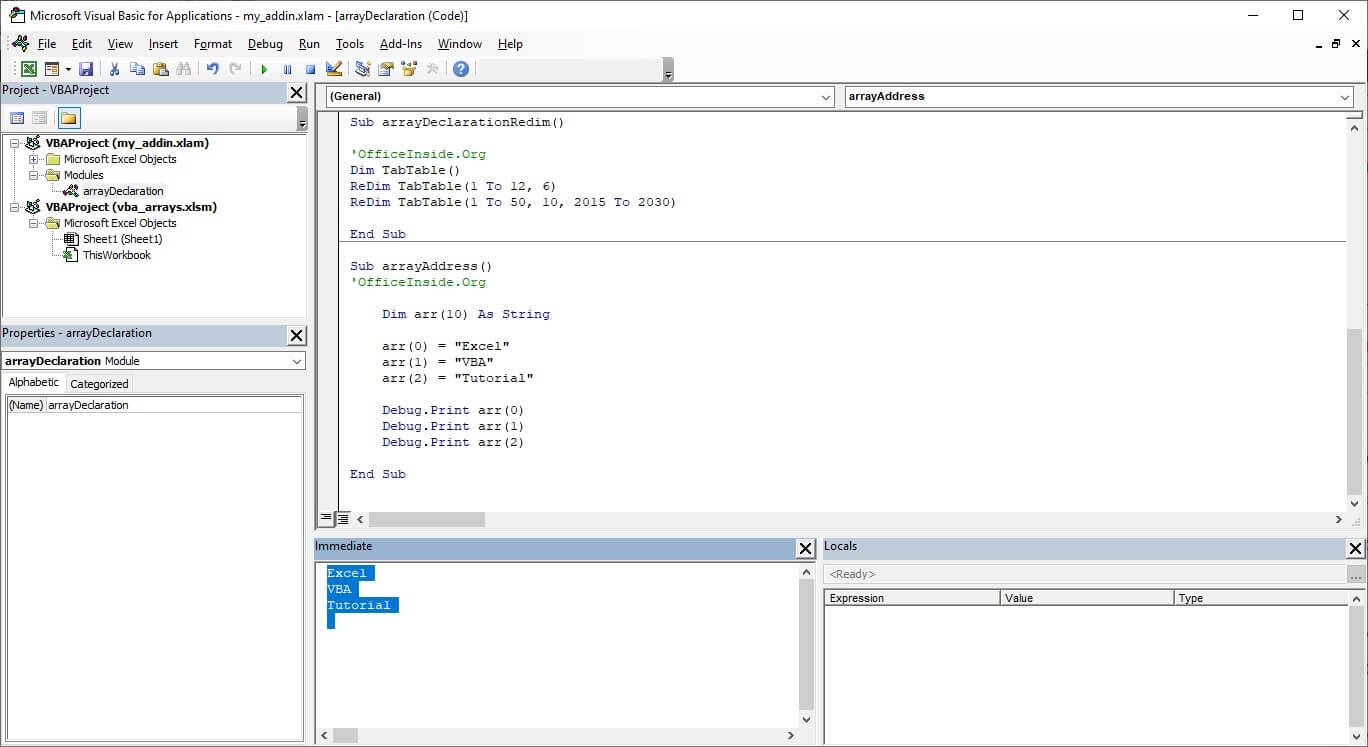Excel VBA Addressing array elements

## Entering data into the Excel VBA array

To enter data into array, you must specify the dimension, and elements of the array, which should be filled. Depending on the declaration method, you select the element of the array by numbered index.

### Enter data into simple VBA Array from code

```Sub arrExample1()

Dim arr(6) As String

`arr(0) = "Sunday" `
`arr(1) = "Monday" `
`arr(2) = "Tuesday" `
`arr(3) = "Wednesday" `
`arr(4) = "Thursday" `
`arr(5) = "Friday" `
`arr(6) = "Saturday"`

`'read `
`Debug.Print arr(4)`
End Sub```Entering data into the Excel VBA array

As you probably noticed, the addressing of arrays declared with the determination of the total number of elements starts from zero. This is the number of the first index of an array. So if you would like to have 10 elements, you should declare an array as follows Name (9).

### Enter data into multidimensional VBA Array from code

If you want to enter data into multidimensional array, you proceed in a similar way by specifying the element according to its indexes relative to the individual dimensions of the table.

```Sub arrayMulti()
'OfficeInside.Org

`'3-dimensional array declaration `
`Dim arr(1 To 31, 1 To 12, 2000 To 2030) `

`'Data enter `
`arr(30, 9, 2020) = WeekdayName(2, False, vbMonday) `

`'Data read `
`MsgBox arr(30, 9, 2020)`
End Sub```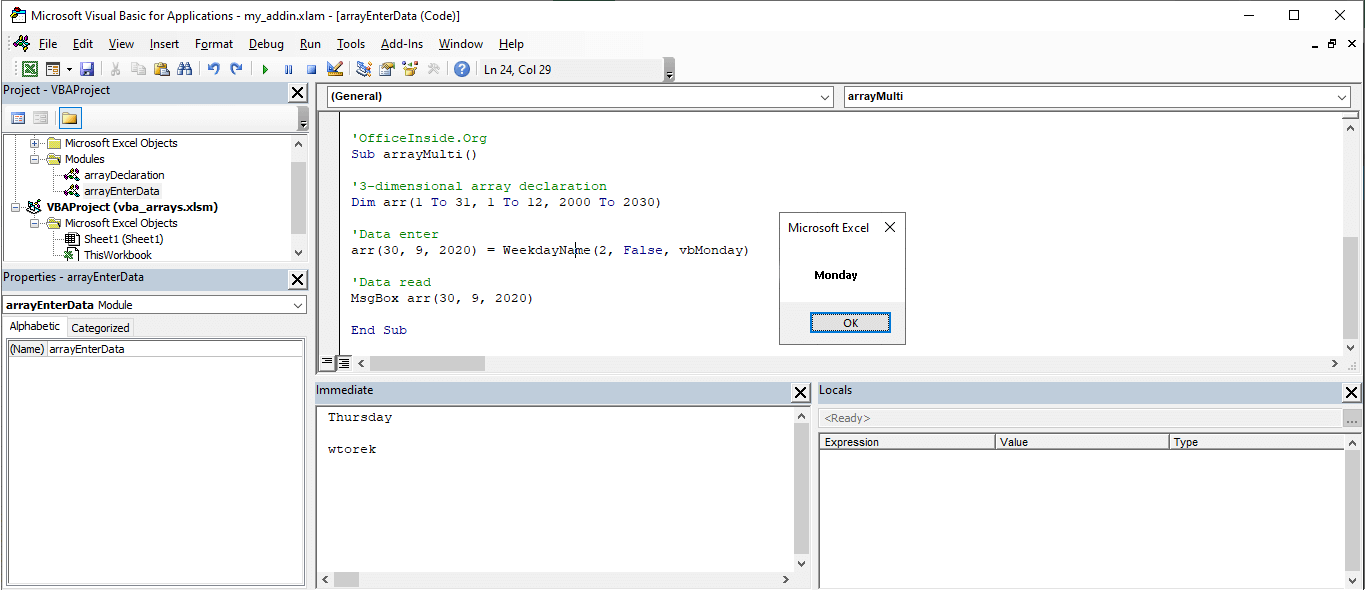Enter data into multidimensional VBA Array from code

### Enter data into VBA Array from sheet or inputBox

Data can also be entered directly from the data sheet or from the VBA InputBox window . How to do it? Below are examples:

From inputBox

```Sub arrayFromInputBox()
'OfficeInside.Org

`'3-dimensional array declaration `
`Dim arr(1 To 10, 1 To 10, 1 To 10) As Variant `

`'Enter data `
`arr(1, 1, 1) = InputBox("Please enter the data to 1,1,1 element") `

`'Read data `
`MsgBox arr(1, 1, 1)`
End Sub```

From Excel Sheet

```Sub arrayFromSheet()

`'Read data from A1 cell `
`Range("A1") = "My Data" `

`'Insert data from into array `
`arr(2, 2, 2) = Range("A1") `

`'Ready data MsgBox `
`arr(2, 2, 2)`
End Sub
```

## Read data from the Excel VBA array

If you want to read data from array, the only thing you should do is address the array element. Data from array can be load to the variable VBA, displayed in the VBA window MsgBox, enter directly into the datasheet. Here is an example:

```Sub arrRead()
'OfficeInside.Org

`'3-dimensional array declaration `
`Dim arr(1 To 10, 1 To 10, 1 To 10) As Variant `

`'Enter data into array `
`arr(1, 1, 1) = InputBox("Enter element: 1,1,1") `

`'Enter data from array to A1-cell `
`Range("A1") = arr(1, 1, 1) `

`'Display data in msgBox window `
`MsgBox arr(1, 1, 1)`
End Sub```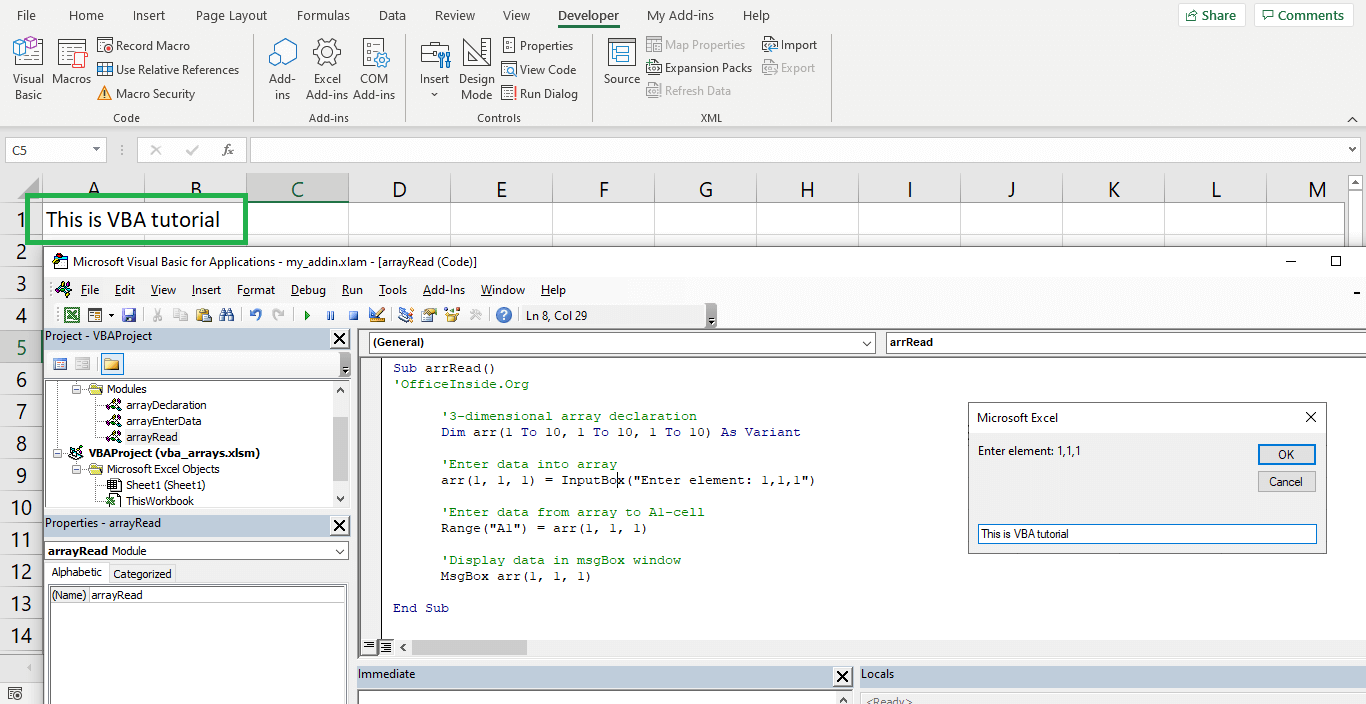Read data from the Excel VBA array

## Application programming with VBA Arrays in Excel

What can we use arrays in VBA? Arrays primarily speed up calculations based on Excel data. You can create complex mathematical models. In the next topic, you will learn additional informations about VBA arrays. You will learn how to create arrays using the Array function . You will learn how to perform bulk operations between arrays and excel data sheet.

# VBA Arrays – information for advanced users

If you are reading this topic, you have previously read the basic information about VBA arrays. In this topic you will learn more advanced informations. Informations about functions that you use with arrays, how to quickly read data from array and how to quickly load them into array.

## VBA Arrays functions: LBound UBound IsArray

In the case of VBA tables, we can use functions relating to the whole table. We can use them to check if our variable is an array. We can also check the subscript and subscript index of the array to check its size. To test the aforementioned functions, create a simple array and fill it with values, remembering not to fill all the elements of the array. The code creating and filling such a table is given below:

```'OfficeInside.Org
Sub arrayFunctions()

Dim arr(9) As String

arr(3) = "Robert Kubica"
arr(6) = "Michael Schumacher"
arr(8) = "Nico Resberg"

Debug.Print IsArray(arr)
Debug.Print LBound(arr)
Debug.Print UBound(arr)

End Sub```

As you can see, we created a 10-element array with indexes from 0 to 9. As you can see, the VBA IsArray function returns True value when your variable is array. The LBound and Ubound functions return indexes elements which are filled. Below you can read extended description.

## Extended description of the array functions

• IsArray – The function returns True when variable is an array.
• LBound (array [, dimension]) – The function returns the lower array index . If you work on multidimensional arrays, the second function argument is used to give the dimension in the array that you want to check. From basic information about VBA arrays, you know that dimensions can have different ranges. If you are working with a multidimensional array, and you don’t complete the argument specifying the dimension, the function returns the index for the first dimension of the array.
• UBound (array [, dimension]) – The function returns the upper array index . If we work on multidimensional arrays, the second function argument is used to give the dimension of the array that you want to check. If you are working with a multidimensional array, and you don’t complete the argument specifying the dimension, the function returns the index for the first dimension of the array.

## Example of LBound and UBound for multidimensional arrays

Here is a short example of the use of the LBound and Ubound functions for two-dimensional and three-dimensional arrays. The dimension is defined by a number from 1 to the number of dimensions of the array. If you do not specify it, the VBA LBound and UBound functions will return index information for the first dimension of the array.

```'OfficeInside.Org
Sub arrayFunctions2()

Dim arr(9, 1 To 5) As String
Dim arr(2, 1 To 5, 18) As String

Debug.Print LBound(arr, 2)
Debug.Print UBound(arr)

Debug.Print LBound(arr)
Debug.Print UBound(arr, 3)

End Sub```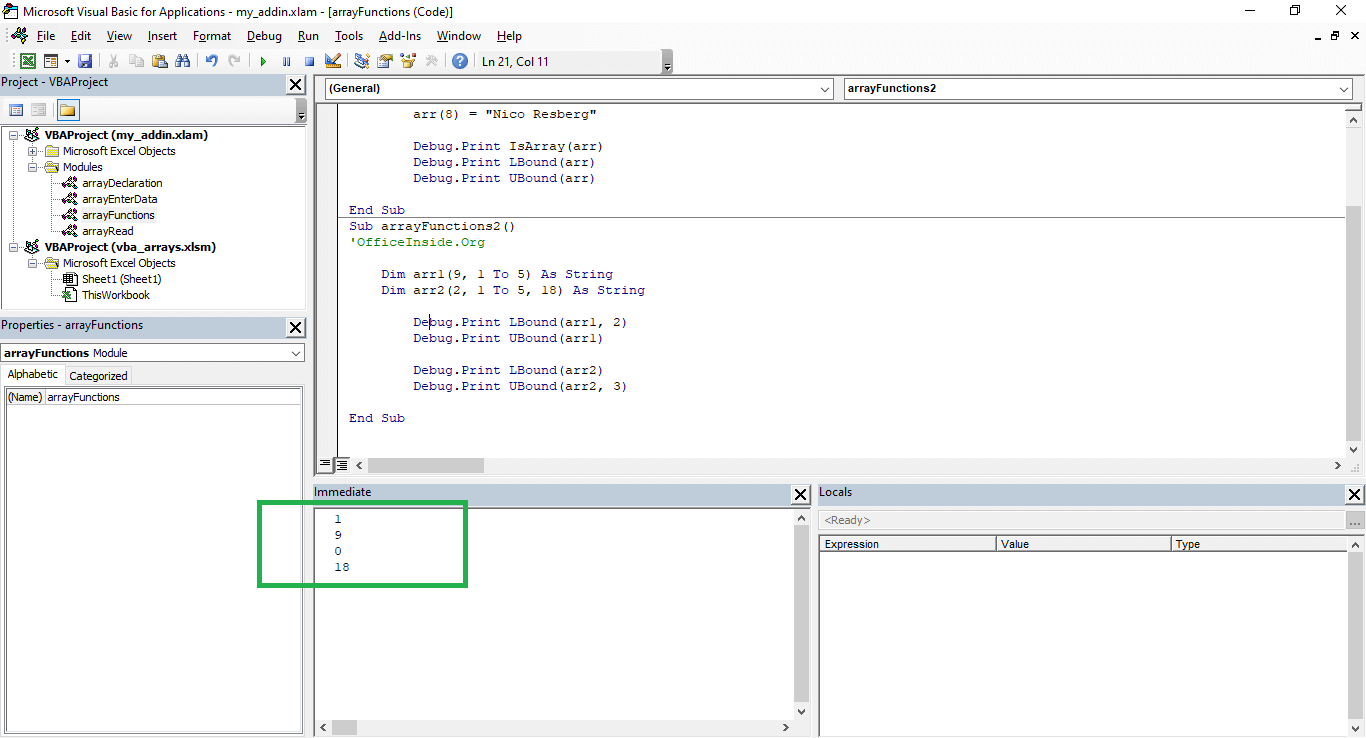Excel VBA tutorial: Example of LBound and UBound for multidimensional arrays

## Creating an array using Array function

Arrays can also be created using the VBA Array function. How to do it? Create a variable with the Variant data type. Then assign values ​​to it in array arguments separated by commas. Here is an example:

```'OfficeInside.Org
Sub arrayFunctionExample()

Dim Countries As Variant
Countries = Array("USA", "Germany", "England", "France")
Debug.Print Countries(0)

Dim Cities As Variant
Cities = Array("Washington", "Berlin", "London", "Paris")
Debug.Print Cities(0)

End Sub```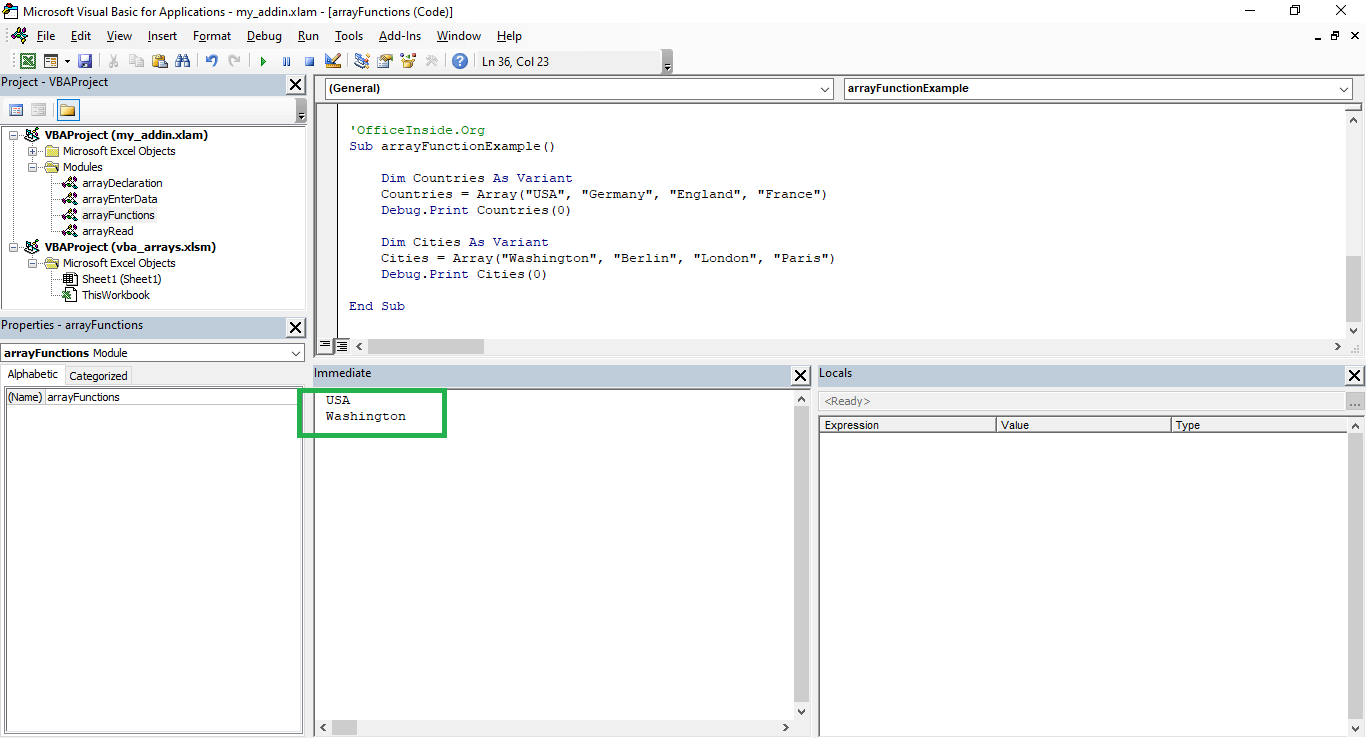VBA Course: Creating an array using Array function

## Fast data load from the worksheet to the VBA array

How to quickly insert data to the VBA array? You can load data to the VBA array in many ways. You can of course use VBA Loop, which will take all elements of the sheet, but this isn’t a quick solution. The easiest way to enter data from an Excel spreadsheet into a two-dimensional array (because the sheet also has two dimensions) is a reference to the range.

In order to practice the file attached to this article, I added a sheet containing data. The following example insert data into array. In this task you need to enter the data table and show data from the second table record. Remember that you need to omit the headers when importing data from the worksheet to the array.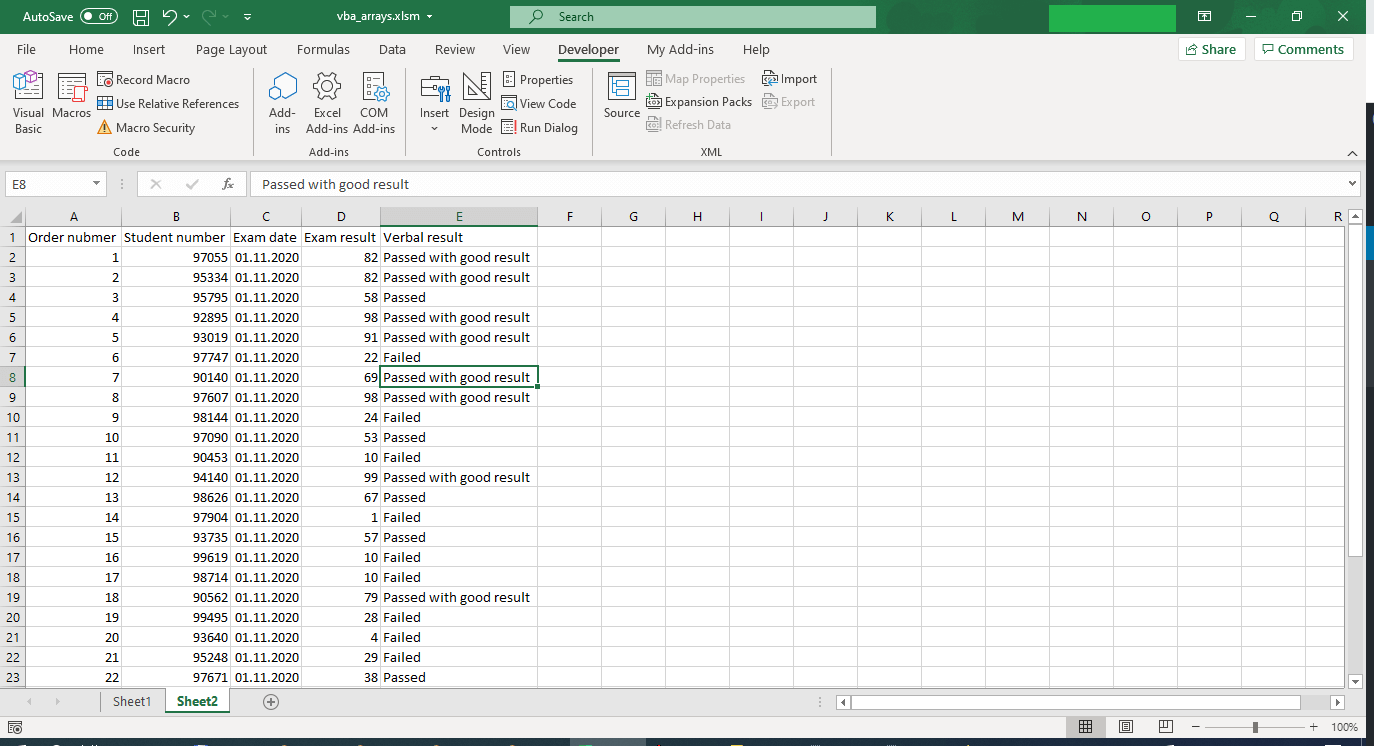Fast data load from the worksheet to the VBA array
```'OfficeInside.Org
Sub sheetToArray()

Dim dataFromSheet As Variant
dataFromSheet = Range("A2:E30")

'check
Debug.Print dataFromSheet(2, 1)
Debug.Print dataFromSheet(2, 2)
Debug.Print dataFromSheet(2, 3)
Debug.Print dataFromSheet(2, 4)
Debug.Print dataFromSheet(2, 5)

End Sub```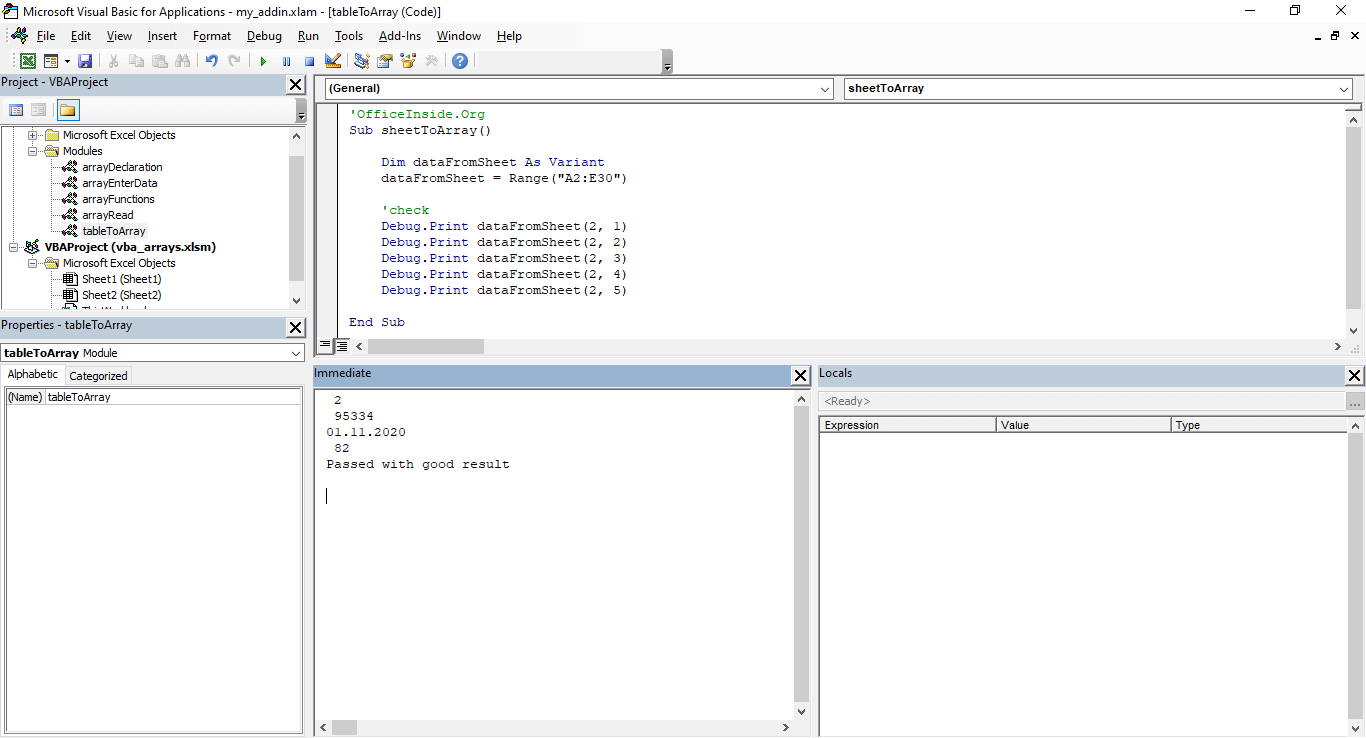VBA Array from excel table

The article is part of the Vba Excel course. The whole course can be found at this link:  VBA course. Below you can find the file with the program code:

## Tasks (You can enter the solution in a comment)

• Create a two-dimensional array, 7 and 12 elements in dimensions. Assign the names of the days of the week and the names of the months to the array elements.
• Create a one-dimensional array. Assign it to years 2000 to 2030 using the Do While loop.